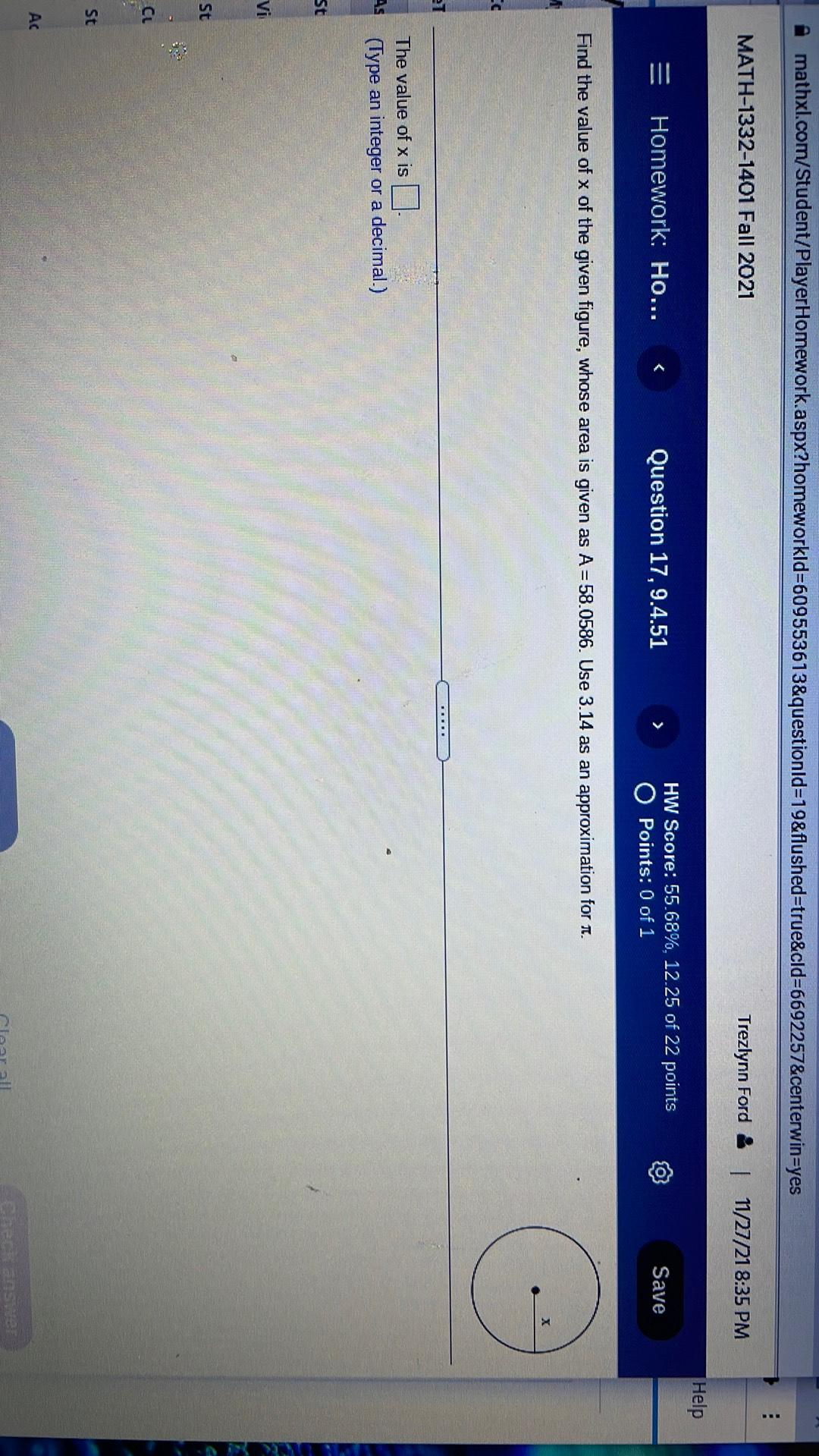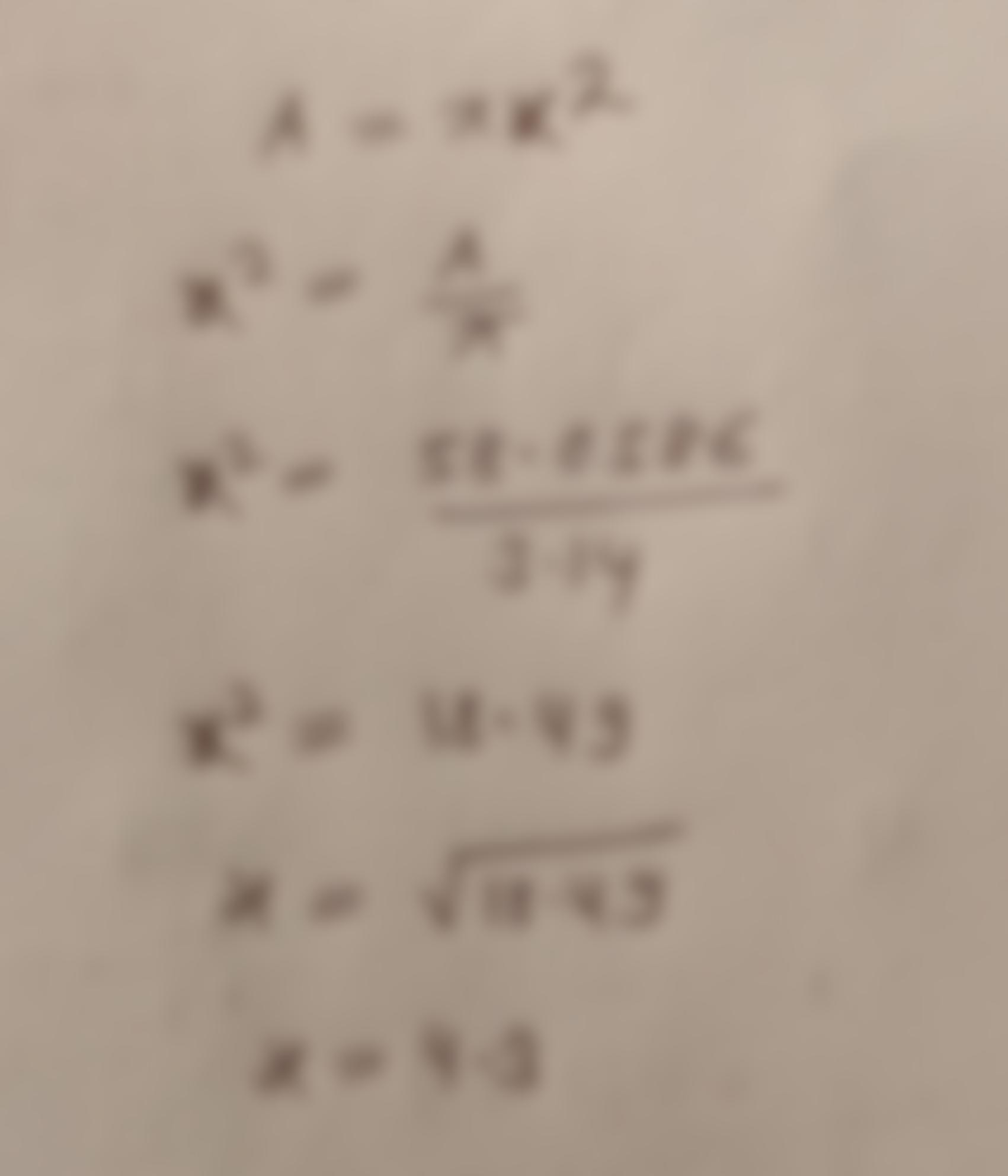Question:

mathxl.com/Student/Player Homework.aspx?homeworkld=609553613&questionid=19&flushed=true&cld=6692257&centerwin=yes MATH-1332-1401mathxl.com/Student/Player Homework.aspx?homeworkld=609553613&questionid=19&flushed=true&cld=6692257&centerwin=yes MATH-1332-1401 Fall 2021 Trezlynn Ford & 1 11/27/21 8:35 PM Help Homework: Ho... Question 17, 9.4.51 HW Score: 55.68%, 12.25 of 22 points O Points: 0 of 1 Save Find the value of x of the given figure, whose area is given as A = 58.0586. Use 3.14 as an approximation for I. EC IB... The value of x is (Type an integer or a decimal.) NA St Vid St CL St AC